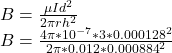## A hollow cylinder with an inner radius of and an outer radius of conducts a 3.0-A current flowing parallel to the axis of the cylinder. If t

Question

A hollow cylinder with an inner radius of and an outer radius of conducts a 3.0-A current flowing parallel to the axis of the cylinder. If the current density is uniform throughout the wire, what is the magnitude of the magnetic field at a point from its center?

in progress 0
3 months 2021-08-17T01:49:07+00:00 1 Answers 4 views 0

1. Complete Question:

A hollow cylinder with an inner radius of 4.0mm and an outer radius of 30mm conducts a 3.0-A current flowing parallel to the axis of the cylinder. If the current density is uniform throughout the wire, what is the magnitude of the magnetic field at a point 12mm from its center?

The magnitude of the magnetic field = 7.24 μT

Explanation:

Inner radius, a = 4.0 mm = 0.004 m

Outer radius, b = 30 mm = 0.03 m

Radius, r = 12 mm = 0.012 m

let h² = b² – a²

h² = 0.03² – 0.004²

h² = 0.000884

Let d² = r² – a²

d² = 0.012² – 0.004²

d² = 0.000128

Current I = 3A

μ = 4π * 10⁻⁷

The magnitude of the magnetic field is given by:B = 7.24 * 10⁻⁶T

B = 7.24 μT